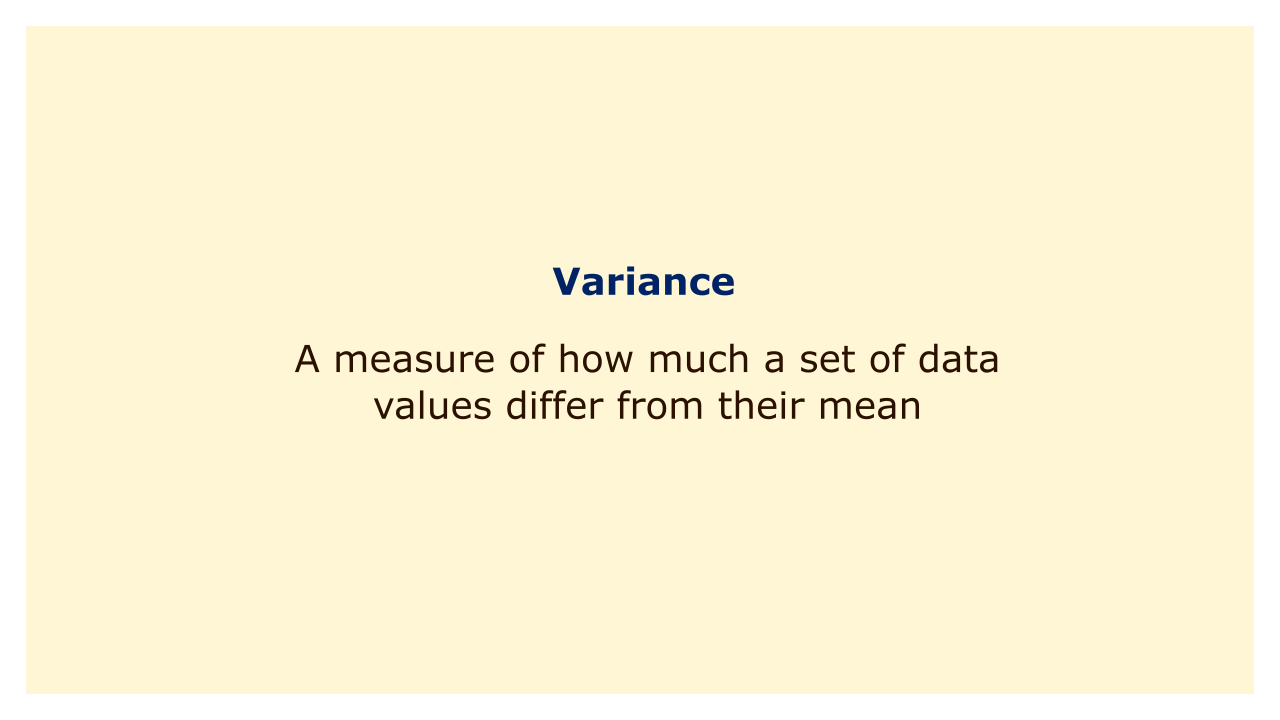# VarianceImage: Moneybestpal.com

### Variance is a measure of how much a set of data values differ from their mean. It is determined by averaging the squared deviations between each data value and the mean. A high variance suggests that the data values are widely dispersed around the mean, whereas a low variance suggests that they are closely grouped.

Variance is a crucial term in statistics because it enables us to assess the accuracy with which a sample represents the population. For instance, if we wanted to estimate the average height of people in a nation, we could measure the heights of a random sample of people. The heights are, however, considerably dissimilar from one another and from the mean if the sample has a significant variation. This can suggest that the population is not adequately represented in the sample, or that the data contains anomalies or inaccuracies.

On the other hand, a low variance indicates that the heights in the sample are comparable to one another and to the mean. This can suggest that the sample is representative of the entire population or that there are no anomalies or data problems.

Additionally, variance can be used to contrast two or more sets of data values. To compare the heights of men and women in a nation, for instance, we may compute the variance for each group and determine which one has a higher or smaller variance. A higher variance indicates that the group is more diverse or variable, whereas a lower variance indicates that the group is more homogeneous or consistent.

Different formulas can be used to calculate variance depending on whether the data values come from a population or a sample. The sum of the squared deviations between each data value and the population mean is multiplied by the quantity of data values, and the result is the population variance, represented by the symbol σ^2. By dividing the total of the squared deviations between each data value and the sample mean by the number of data values minus one, the sample variance, represented by the symbol s^2, is computed. To account for bias and make the sample variance a fair estimate of the population variance, one is subtracted from the denominator.

The standard deviation and range of dispersion metrics are connected to variance. Standard deviation, which calculates how much data values depart from their mean in either direction, is just variance's square root. In order to determine how far apart the data values are from one another, range is defined as the difference between the maximum and least data values. We can characterize, examine, and draw inferences from data sets using all of these metrics.
Tags